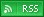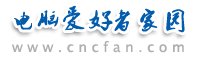电脑爱好者，提供IT资讯信息及各类编程知识文章介绍，欢迎大家来本站学习电脑知识。 最近更新 | 联系我们站内搜索：

# 使用VBScript操作Html复选框（CheckBox）控件

OK，切入主题，现介绍一下：

'*************************************************
'<函数名>
' gfIs_ArrayTest(obj)
'<功能>
' 判断选定的CheckBox的是一个还是一个以上
'<参数>
' CheckBox对象名
'<返回值>
' true
' false
'<备注>
' Created on Mar.15th.2004 by AITD
'*************************************************
function gfIs_ArrayTest(obj)
on error resume next
if obj.type = "flag" then
gfIs_ArrayTest = True
else
gfIs_ArrayTest = False
end if
end function

'******************************************************
'<函数名>
' gfChkCheckbox()
'<功能>
' 检查画面CheckBox的选择是否符合要求
'<参数>
' obj: 对应的checkbox控件
' flag: 检查规则：0 可有多个被选中
' 1 只能有一个被选中
' name: 提示用户那个控件报错的信息
'<返回值>
' true
' false
'<备注>
' Created on Mar.15th.2004 by AITD
'******************************************************
function gfChkCheckbox(obj,flag,name)
Dim i
Dim j

gfChkCheckbox = false
i = 0
j = 0
'若对象不存在
if isnull(obj) then
msgbox "请选择一个 " + name + "。" '请选择一个对象
gfChkCheckbox = false
exit function
end if

'对象只有一个
if not gfIs_ArrayTest(obj) then
if obj.checked = false then
msgbox "请选择一个 " + name + "。" '请选择一个对象
gfChkCheckbox = false
exit function
else
gfChkCheckbox = true
exit function
end if
end if

'若有多个对象存在

for i = 0 to (obj.length - 1)
if obj(i).type = "checkbox" then
if obj(i).checked = true then
j = j + 1
end if
end if
next

if j = 0 then
msgbox "请选择 " + name + "。" '请选择一个对象
gfChkCheckbox = false
exit function
end if
if j = 1 then
gfChkCheckbox = true
exit function
end if
if j > 1 then
if flag = 1 then
msgbox "只能选择一个 " + name + "。" '只能选择一个对象
gfChkCheckbox = false
exit function
else
gfChkCheckbox = true
exit function
end if
end if

end function

'*************************************************
'<函数名>
' gfGetCheckBoxValue(obj)
'<功能>
' 取得选定的CheckBox的值
'<参数>
' CheckBox对象名
'<返回值>
' 选定的CheckBox的值
'<备注>
' Created on Mar.15th.2004 by AITD
'*************************************************
function gfGetCheckBoxValue(obj)

dim strValue
dim intCounter
dim i

strValue = ""
intCounter = 0

'如果对象不存在
if isnull(obj) then
gfGetCheckBoxValue = strValue
exit function
end if

'如果对象为一个
if not gfIs_ArrayTest(obj) then
if obj.checked = false then
gfGetCheckBoxValue = strValue
exit function
else
gfGetCheckBoxValue = obj.value
exit function
end if
end if

'如果对象为多个
for i = 0 to obj.length - 1
if obj(i).checked = true then
if intCounter > 0 then
strValue = strValue & "^|^" & CStr(obj(i).value)
else
strValue = CStr(obj(i).value)
end if
intCounter = intCounter + 1
end if
next

gfGetCheckBoxValue = strValue
end function

function sIsChkBoxExist()
on error resume next
err.clear
if isEmpty(document.frmOrderList.chkOrder) then
end if
if err.number <> 0 then
else
sIsChkBoxExist=true
end if
err.clear
end function

- EOM -
 Vbscript热门文章排行 网站赞助商